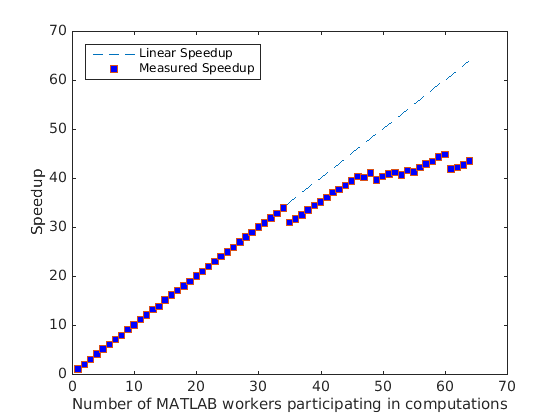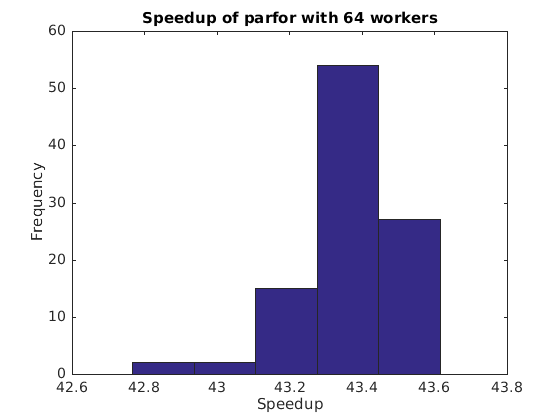Main Content

# Simple Benchmarking of PARFOR Using Blackjack

This example benchmarks the `parfor` construct by repeatedly playing the card game of blackjack, also known as 21. We use `parfor` to play the card game multiple times in parallel, varying the number of MATLAB® workers, but always using the same number of players and hands.

Related examples:

### Parallel Version

The basic parallel algorithm uses the `parfor` construct to execute independent passes through a loop. It is a part of the MATLAB® language, but behaves essentially like a regular `for`-loop if you do not have access to the Parallel Computing Toolbox™ product. Thus, our initial step is to convert a loop of the form

```for i = 1:numPlayers S(:, i) = playBlackjack(); end ```

into the equivalent `parfor` loop:

```parfor i = 1:numPlayers S(:, i) = playBlackjack(); end ```

We modify this slightly by specifying an optional argument to `parfor`, instructing it to limit to `n` the number of workers it uses for the computations. The actual code is as follows:

```dbtype pctdemo_aux_parforbench ```
```1 function S = pctdemo_aux_parforbench(numHands, numPlayers, n) 2 %PCTDEMO_AUX_PARFORBENCH Use parfor to play blackjack. 3 % S = pctdemo_aux_parforbench(numHands, numPlayers, n) plays 4 % numHands hands of blackjack numPlayers times, and uses no 5 % more than n MATLAB(R) workers for the computations. 6 7 % Copyright 2007-2009 The MathWorks, Inc. 8 9 S = zeros(numHands, numPlayers); 10 parfor (i = 1:numPlayers, n) 11 S(:, i) = pctdemo_task_blackjack(numHands, 1); 12 end ```

### Check the Status of the Parallel Pool

We will use the parallel pool to allow the body of the `parfor` loop to run in parallel, so we start by checking whether the pool is open. We will then run the benchmark using anywhere between 2 and `poolSize` workers from this pool.

```p = gcp; if isempty(p) error('pctexample:backslashbench:poolClosed', ... ['This example requires a parallel pool. ' ... 'Manually start a pool using the parpool command or set ' ... 'your parallel preferences to automatically start a pool.']); end poolSize = p.NumWorkers; ```

### Run the Benchmark: Weak Scaling

We time the execution of our benchmark calculations using 2 to `poolSize` workers. We use weak scaling, that is, we increase the problem size with the number of workers.

```numHands = 2000; numPlayers = 6; fprintf('Simulating each player playing %d hands.\n', numHands); t1 = zeros(1, poolSize); for n = 2:poolSize tic; pctdemo_aux_parforbench(numHands, n*numPlayers, n); t1(n) = toc; fprintf('%d workers simulated %d players in %3.2f seconds.\n', ... n, n*numPlayers, t1(n)); end ```
```Simulating each player playing 2000 hands. 2 workers simulated 12 players in 10.81 seconds. 3 workers simulated 18 players in 10.67 seconds. 4 workers simulated 24 players in 10.57 seconds. 5 workers simulated 30 players in 10.57 seconds. 6 workers simulated 36 players in 10.71 seconds. 7 workers simulated 42 players in 10.63 seconds. 8 workers simulated 48 players in 10.87 seconds. 9 workers simulated 54 players in 10.54 seconds. 10 workers simulated 60 players in 10.73 seconds. 11 workers simulated 66 players in 10.58 seconds. 12 workers simulated 72 players in 10.68 seconds. 13 workers simulated 78 players in 10.56 seconds. 14 workers simulated 84 players in 10.89 seconds. 15 workers simulated 90 players in 10.62 seconds. 16 workers simulated 96 players in 10.63 seconds. 17 workers simulated 102 players in 10.70 seconds. 18 workers simulated 108 players in 10.70 seconds. 19 workers simulated 114 players in 10.79 seconds. 20 workers simulated 120 players in 10.72 seconds. 21 workers simulated 126 players in 10.74 seconds. 22 workers simulated 132 players in 10.75 seconds. 23 workers simulated 138 players in 10.74 seconds. 24 workers simulated 144 players in 10.72 seconds. 25 workers simulated 150 players in 10.74 seconds. 26 workers simulated 156 players in 10.76 seconds. 27 workers simulated 162 players in 10.74 seconds. 28 workers simulated 168 players in 10.72 seconds. 29 workers simulated 174 players in 10.76 seconds. 30 workers simulated 180 players in 10.69 seconds. 31 workers simulated 186 players in 10.76 seconds. 32 workers simulated 192 players in 10.76 seconds. 33 workers simulated 198 players in 10.79 seconds. 34 workers simulated 204 players in 10.74 seconds. 35 workers simulated 210 players in 12.12 seconds. 36 workers simulated 216 players in 12.19 seconds. 37 workers simulated 222 players in 12.19 seconds. 38 workers simulated 228 players in 12.14 seconds. 39 workers simulated 234 players in 12.15 seconds. 40 workers simulated 240 players in 12.18 seconds. 41 workers simulated 246 players in 12.18 seconds. 42 workers simulated 252 players in 12.14 seconds. 43 workers simulated 258 players in 12.24 seconds. 44 workers simulated 264 players in 12.25 seconds. 45 workers simulated 270 players in 12.23 seconds. 46 workers simulated 276 players in 12.23 seconds. 47 workers simulated 282 players in 12.55 seconds. 48 workers simulated 288 players in 12.52 seconds. 49 workers simulated 294 players in 13.24 seconds. 50 workers simulated 300 players in 13.28 seconds. 51 workers simulated 306 players in 13.36 seconds. 52 workers simulated 312 players in 13.53 seconds. 53 workers simulated 318 players in 13.98 seconds. 54 workers simulated 324 players in 13.90 seconds. 55 workers simulated 330 players in 14.29 seconds. 56 workers simulated 336 players in 14.23 seconds. 57 workers simulated 342 players in 14.25 seconds. 58 workers simulated 348 players in 14.32 seconds. 59 workers simulated 354 players in 14.26 seconds. 60 workers simulated 360 players in 14.34 seconds. 61 workers simulated 366 players in 15.60 seconds. 62 workers simulated 372 players in 15.75 seconds. 63 workers simulated 378 players in 15.79 seconds. 64 workers simulated 384 players in 15.76 seconds. ```

We compare this against the execution using a regular `for`-loop in MATLAB®.

```tic; S = zeros(numHands, numPlayers); for i = 1:numPlayers S(:, i) = pctdemo_task_blackjack(numHands, 1); end t1(1) = toc; fprintf('Ran in %3.2f seconds using a sequential for-loop.\n', t1(1)); ```
```Ran in 10.70 seconds using a sequential for-loop. ```

### Plot the Speedup

We compare the speedup using `parfor` with different numbers of workers to the perfectly linear speedup curve. The speedup achieved by using `parfor` depends on the problem size as well as the underlying hardware and networking infrastructure.

```speedup = (1:poolSize).*t1(1)./t1; fig = pctdemo_setup_blackjack(1.0); fig.Visible = 'on'; ax = axes('parent', fig); x = plot(ax, 1:poolSize, 1:poolSize, '--', ... 1:poolSize, speedup, 's', 'MarkerFaceColor', 'b'); t = ax.XTick; t(t ~= round(t)) = []; % Remove all non-integer x-axis ticks. ax.XTick = t; legend(x, 'Linear Speedup', 'Measured Speedup', 'Location', 'NorthWest'); xlabel(ax, 'Number of MATLAB workers participating in computations'); ylabel(ax, 'Speedup'); ```### Measure the Speedup Distribution

To get reliable benchmark numbers, we need to run the benchmark multiple times. We therefore run the benchmark multiple times for `poolSize` workers to allow us to look at the spread of the speedup.

```numIter = 100; t2 = zeros(1, numIter); for i = 1:numIter tic; pctdemo_aux_parforbench(numHands, poolSize*numPlayers, poolSize); t2(i) = toc; if mod(i,20) == 0 fprintf('Benchmark has run %d out of %d times.\n',i,numIter); end end ```
```Benchmark has run 20 out of 100 times. Benchmark has run 40 out of 100 times. Benchmark has run 60 out of 100 times. Benchmark has run 80 out of 100 times. Benchmark has run 100 out of 100 times. ```

### Plot the Speedup Distribution

We take a close look at the speedup of our simple parallel program when using the maximum number of workers. The histogram of the speedup allows us to distinguish between outliers and the average speedup.

```speedup = t1(1)./t2*poolSize; clf(fig); ax = axes('parent', fig); hist(speedup, 5); a = axis(ax); a(4) = 5*ceil(a(4)/5); % Round y-axis to nearest multiple of 5. axis(ax, a) xlabel(ax, 'Speedup'); ylabel(ax, 'Frequency'); title(ax, sprintf('Speedup of parfor with %d workers', poolSize)); m = median(speedup); fprintf(['Median speedup is %3.2f, which corresponds to '... 'efficiency of %3.2f.\n'], m, m/poolSize); ```
```Median speedup is 43.37, which corresponds to efficiency of 0.68. ```## Support평가판 신청